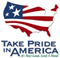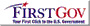Publications—Techniques and Methods

# Kendall-Theil Robust Line (KTRLine—version 1.0)—A Visual Basic Program for Calculating and Graphing Robust Nonparametric Estimates of Linear-Regression Coefficients Between Two Continuous Variables

By Gregory E. Granato

### Chapter 7 Section A, Statistical Analysis, Book 4, Hydrologic Analysis and Interpretation

U.S. Geological Survey Techniques and Methods 4-A7

The body of the report is available in PDF Format: Screen resolution (967 KB) | High resolution (1.5 MB)

Software Information and Installation

## Abstract

The Kendall-Theil Robust Line software (KTRLine—version 1.0) is a Visual Basic program that may be used with the Microsoft Windows operating system to calculate parameters for robust, nonparametric estimates of linear-regression coefficients between two continuous variables. The KTRLine software was developed by the U.S. Geological Survey, in cooperation with the Federal Highway Administration, for use in stochastic data modeling with local, regional, and national hydrologic data sets to develop planning-level estimates of potential effects of highway runoff on the quality of receiving waters. The Kendall-Theil robust line was selected because this robust nonparametric method is resistant to the effects of outliers and nonnormality in residuals that commonly characterize hydrologic data sets. The slope of the line is calculated as the median of all possible pairwise slopes between points. The intercept is calculated so that the line will run through the median of input data. A single-line model or a multisegment model may be specified.

The program was developed to provide regression equations with an error component for stochastic data generation because nonparametric multisegment regression tools are not available with the software that is commonly used to develop regression models. The Kendall-Theil robust line is a median line and, therefore, may underestimate total mass, volume, or loads unless the error component or a bias correction factor is incorporated into the estimate. Regression statistics such as the median error, the median absolute deviation, the prediction error sum of squares, the root mean square error, the confidence interval for the slope, and the bias correction factor for median estimates are calculated by use of nonparametric methods. These statistics, however, may be used to formulate estimates of mass, volume, or total loads.

The program is used to read a two- or three-column tab-delimited input file with variable names in the first row and data in subsequent rows. The user may choose the columns that contain the independent (X) and dependent (Y) variable. A third column, if present, may contain metadata such as the sample-collection location and date. The program screens the input files and plots the data. The KTRLine software is a graphical tool that facilitates development of regression models by use of graphs of the regression line with data, the regression residuals (with X or Y), and percentile plots of the cumulative frequency of the X variable, Y variable, and the regression residuals. The user may individually transform the independent and dependent variables to reduce heteroscedasticity and to linearize data. The program plots the data and the regression line. The program also prints model specifications and regression statistics to the screen. The user may save and print the regression results. The program can accept data sets that contain up to about 15,000 XY data points, but because the program must sort the array of all pairwise slopes, the program may be perceptibly slow with data sets that contain more than about 1,000 points.

Abstract

Introduction

Statistical Theory and Governing Equations

Parametric Regression

Nonparametric Regression

Governing Equations for Kendall-Theil Robust Line Regression

Slope

Intercept

Residual Error

Regression Statistics

The Bias Correction Factor

Cunnane Plotting Position Formula

The Point of Convergence for Multisegment Models

Development of a Regression Model

Use of the KTRLine Software

Installation and Removal

Creating an Input-Data File

Input-Data Specification Form

Open and Test Input File

Input-Data Specification Options

Filter Input Data

Process Input Data

Graph Data

Exit Program

Interpretive Graphing and Model Specification Form

Graphical-Display Interface

Data-Identification Tool

Multisegment Regression-Model Output Form

KTRLine Output-File Format

Program Performance and Numerical Limitations

Summary and Conclusions

Acknowledgments

References Cited

## Figures

1. Diagram showing simplified relations between increasing discharge and the concentration of water-quality constituents in a stream or river during base flow and runoff events
2. Graph showing the effect of one high-leverage outlier on estimates of ordinary least-squares regression statistics

3–6. Diagrams showing—

 3 The manual method of determining the median slope. 4 The effect of ties in the independent variable on the number of finite slopes that may be calculated for the Kendall-Theil Robust Line and the representativeness of the median of finite slopes for indicating relations between the X and Y variables. 5 The ladder of powers for use in transforming the independent (X) and(or) dependent (Y) variables to improve a regression model. 6 The bulging rule for transforming curvature to linearity.

7–16. Screen images showing—

 7 Example of the Kendall-Theil Robust Line Input-Data Specification Form as it appears when the user is preparing to graph the data. 8 Example of the Kendall-Theil Robust Line Interpretive Graphing and Model Specification Form with the plot menu selected. 9 Example of the Kendall-Theil Robust Line Interpretive Graphing and Model Specification Form demonstrating the use of the X-axis range tool and the data-point size selector. 10 Example of the Kendall-Theil Robust Line data-identification tool message box. 11 Example of the Kendall-Theil Robust Line Interpretive Graphing and Model Specification Form with a residual plot selected. 12 Example of the Kendall-Theil Robust Line Interpretive Graphing and Model Specification Form with a probability plot of the residuals and their ranked percentiles. 13 Example of the Kendall-Theil Robust Line Interpretive Graphing and Model Specification Form graphics and analysis screen with the transformation tab-strip menu selected 14 Example of the Kendall-Theil Robust Line Interpretive Graphing and Model Specification Form graphics and analysis screen with the specify (multisegment model) tab-strip menu selected. 15 Example of a two-segment model plotted on the Kendall-Theil Robust Line Interpretive Graphing and Model Specification Form 16 Example of the Kendall-Theil Robust Line multisegment model results screen
 17 Text box showing an example of a Kendall-Theil Robust Line output file including A, information about the analysis and results of the preliminary regression line and information about the results of a regression of the log-transformed data; and B, information about the results of a multisegment regression of the log-transformed data. 18 Graph showing relations between the number of samples in the input-data set and processing time in seconds from experiments with four different computers.

#### Suggested citation: Granato, G.E., 2006, Kendall-Theil Robust Line (KTRLine—version 1.0)—A visual basic program for calculating and graphing robust nonparametric estimates of linear-regression coefficients between two continuous variables: Techniques and Methods of the U.S. Geological Survey, book 4, chap. A7, 31 p.

For further information, write to:

Director,
USGS Massachusetts–Rhode Island Water Science CenterU.S. Department of the Interior | U.S. Geological Survey